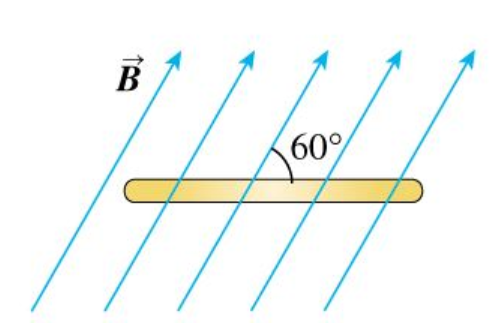# Problem: A flat, circular, steel loop of radius 75 cm is at rest in a uniform magnetic field, as shown in an edge-on view in the figure (Figure 1). The field is changing with time, according to B(t)=(1.4T)e^(−(0.057s−1)t.)Part A: Find the emf induced in the loop as a function of time (assume t is in seconds).Part B: When is the induced emf equal to 1/10 of its initial value?

###### FREE Expert Solution

Induced emf in the loop:

$\overline{){\mathbf{\epsilon }}{\mathbf{=}}{\mathbf{-}}{\mathbf{A}}{\mathbf{c}}{\mathbf{o}}{\mathbf{s}}{\mathbf{\theta }}\frac{\mathbf{d}\mathbf{B}\mathbf{\left(}\mathbf{t}\mathbf{\right)}}{\mathbf{d}\mathbf{t}}}$

Part A

A = πr2

The angle between the magnetic field and normal to the steel is θ = 90° - 60° = 30°

93% (168 ratings)###### Problem Details

A flat, circular, steel loop of radius 75 cm is at rest in a uniform magnetic field, as shown in an edge-on view in the figure (Figure 1). The field is changing with time, according to B(t)=(1.4T)e^(−(0.057s−1)t.)Part A: Find the emf induced in the loop as a function of time (assume t is in seconds).

Part B: When is the induced emf equal to 1/10 of its initial value?